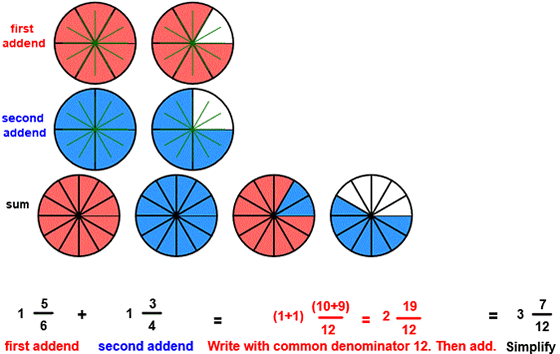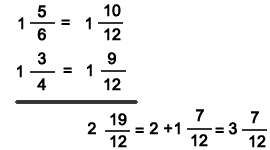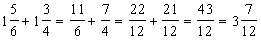CORRECT:
ATTEMPTS:
SCORE:
PERCENT
WHOLE
NUMERATOR
DENOMINATOR

# ADD UNLIKE MIXED FRACTIONS INSTRUCTION

Follow the directions in the dialog box after pressing the <START> button. The <EXPLAIN> button may be pressed after you enter the second addend to see how to do the example.This program is similar to the previous program ADD UNLIKE FRACTIONS except that the addends may be mixed numbers and the sum must be written as a mixed number and in lowest terms.

See the program MIXED NUMBERS for information on writing fractions in mixed form.

See the program RENAME TO LOWEST TERMS for information on writing fractions in lowest terms.

The above image shows a first addend of 1 56 in red and a second addend of 1 34 in blue. The least common denominator of 6 and 4 is 12 and is shown in both addends. So the first addend 1 56 also shows 1 1012 and the second addend 1 34 also shows 1 912.

Now that both addends are written with a common denominator the numerators can be combined for the sum. This is shown in the sum image. Notice how in the sum image that the first addend in red and the second addend in blue are combined for a sum of 3 712.

The math section of the illustration shows that the addends are written with a common denominator 12. The numerators 10 and 9 are then added for a numerator of 19 in the sum. The 19/12 in the sum can be simplified to 1 712 in mixed form. The 1 in 1 712 is added to 2 for a simplified sum of 3 712. The sum must be entered in lowest terms. Although 2 1712 is correct only 3 712 is acceptable for the simplest form of the sum.

You may prefer to work vertically:Or you may prefer to write each addend in fraction form first:In many examples, the sum may be found by visually combining the two addends.

In the above example, the sum (3 712) must be entered as a mixed number and in lowest terms.

For more instruction on adding fractions go to How To Add Fractions.

After you enter the sum you may press the <REPORT> button. The report will ask for your name but you may submit a code for your name. This report will give the same results as on the dialog box. The report may be printed or e-mailed.